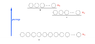# Can Laser be generated with only rapid decay levels?

• genxium

#### genxium

I'm new to laser technology and learning it from some lecture videos and a textbook "Optics & Lasers".

When I came to the light amplifier part, I got stuck in understanding the difference between "rapid decay" and "metastable state" -- what I can recite now is that "rapid decay" cannot generate laser emission while "metastable state" can.

Basically I understand the Professor's description on why a 2-level system is not capable of laser emission in this lecture video (starting from around 05:00) : https://www.youtube.com/watch?v=Re07gHGEZNo

However, what if I have a 3-level system just like the Ruby Laser (depicted in the attachment) but all inter-level transitions are "rapid decay"? Can I generate laser with such a system?

To be specific, if I want to use some material to make a light amplifier and I have:

• 3 energy levels a, b, c where a is the lowest level and b is the highest with the material;
• $n_a, \; n_b, \; n_c$ indicate the number of atoms at each level in the amplifier respectively;
• the amplifier starts with $n_a = N, \; n_b=n_c=0$ where $N$ is an integer;
• atoms at level a will be continually pumped to level b, and I have an infinite energy pump (does the power of the pump matter here?)
• atoms at level b will decay rapidly (generally in nano-seconds as said in the lecture video) to level c
• atoms at level c $ALSO$ decay rapidly to level a!

Can I generate laser with this setting? I expect a continuous rapid decay between level b and level c would occur and laser would be generated by that.

I also have another question with my assumed setting, may I achieve the situation that $n_c > n_a$ at some time? Will "rapid decay" occur when $0< n_c < n_a$ or is "rapid decay" independent of population difference?

Any help will be appreciated :)

#### Attachments

•Screen Shot 2013-09-01 at 9.23.40 PM.png
9.4 KB · Views: 517
Last edited:
You can lase if level c is being filled faster than it is being depleted ... that is, when you solve the "rate master equation" for this three level system you can also determine the pumping rate required for lasing. If level c decays "too fast" then the system won't lase.

It will be instructive to first solve for the two level system ... for a detailed analysis see Siegman's classic text: "Lasers".

Hi UltrafastPED, thank you for the quick reply :)

I'm not sure if I understand your explanation correctly, would you please give me some detailed information? Like is pumping rate important and what do you mean by "rate master equation"? I googled it but what I saw were mostly "XXX Master Equation", can you give me a link to the reference?

They are a set of simultaneous linear differential linear equations that are coupled by the rates of energy transfer between the available modes. You can find many references via Google Scholar.

Shall I search for keyword "Rate Master Equations" ? I did it in Google scholar but there're too many items and I'm not capable of distinguishing the useful ones :( Is it "Quantum Master Equation(s)" or "Master Equation for Atom"? They're the most close items I can see.

Advice: when Google provides too many hits, add more qualifiers. In this case "laser" and "Derivation". This gives
"Phys. Rev. A 9, 2686–2697 (1974)
Modifications to the Scully-Lamb laser master equation"
http://pra.aps.org/abstract/PRA/v9/i6/p2686_1

The references at the end of this paper will lead you back to review papers or the original discussions.

You actually derive a new set of equations for each practical laser design, though the 3-level systems will look similar. You will want to focus on the semi-classical versions until they are clear. Then you can look at the quantum optical/density matrix methods for an advanced study ... preferably in graduate school!

Thank you very much! It's a great help for me :)

I'll read the materials though the equations all look foreign to me -- seems it's time to do some maths again.

Generally, as long as the decay from b to c is slower than from c to a, then there will be a build up of atoms in the c energy state and eventually, a population inversion (and hence lasing).

Beware though, that for a 3 level system, there will be saturation effects (particularly so if the transition from c to a is fast) and so using the rate equations mentioned by UltrafastPED is advisable.

Claude.